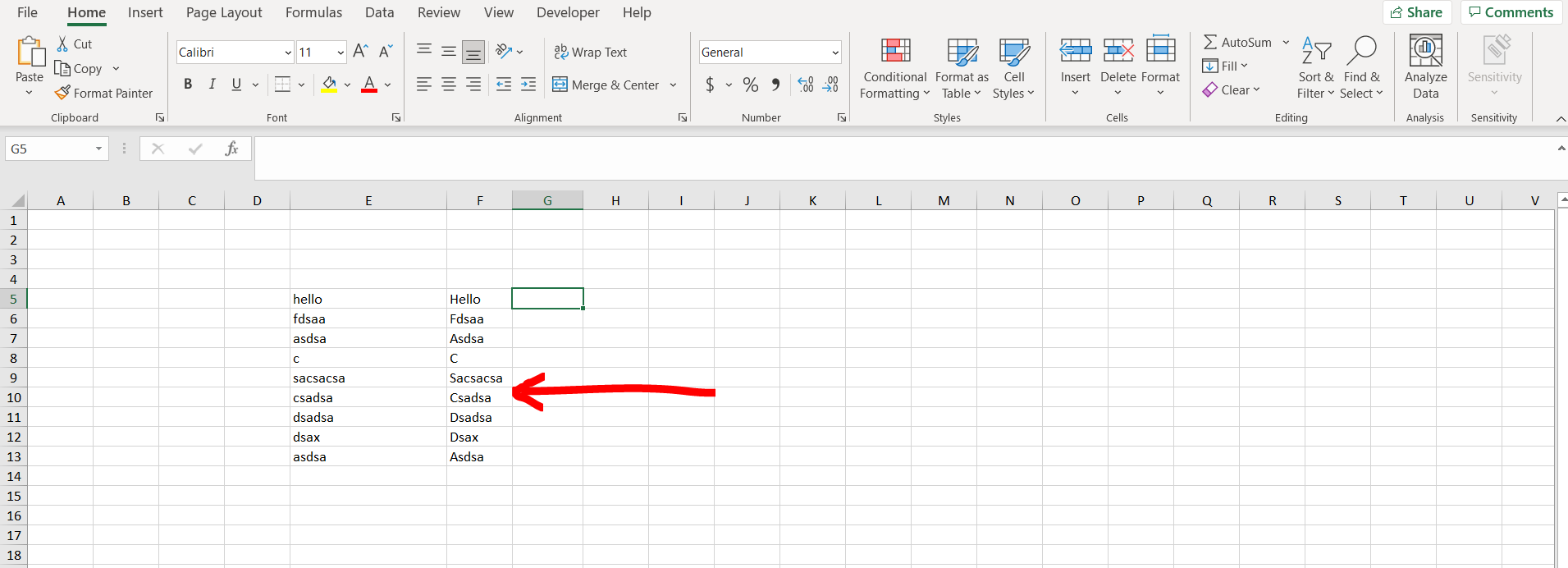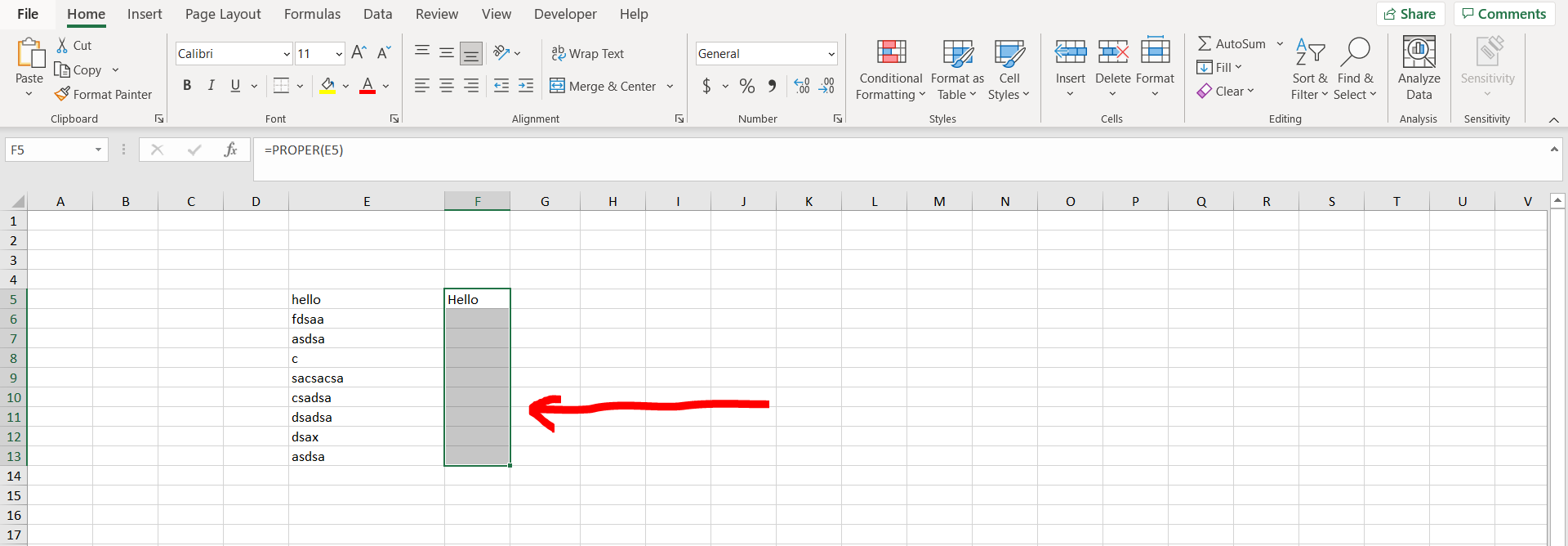# How to drag formula in ExcelExcel offers a very interesting way to drag formula. We can cater to this problem statement by copying the formula to the selection. We can perform the below mentioned way to drag formula in excel:

### Step 1 – Excel workbook with a column with some text– Open the desired Excel workbook which contains a column populated with some text, and adjacent to that write a formula corresponding to one cell.

### Step 2 – Entering the formula– Now copy the cell with the formula and select the number of cells till where you want to carry the formula, and then paste the copied cell.

### Step 3 – Formula dragged– We can see the formula has been dragged till our selection